Courses

# CBSE Class 2 Maths Worksheet- What is Long, What is Round? Notes | EduRev

## Mathematics (Maths) for Class 2

Created by: Yashina Kapoor

## Class 2 : CBSE Class 2 Maths Worksheet- What is Long, What is Round? Notes | EduRev

The document CBSE Class 2 Maths Worksheet- What is Long, What is Round? Notes | EduRev is a part of the Class 2 Course Mathematics (Maths) for Class 2.
All you need of Class 2 at this link: Class 2

Q.1. Circle the 3D shapes that stack.
(i)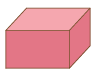(ii)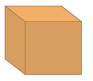(iii)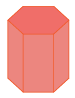(iv)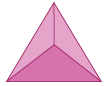(v)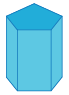(vi)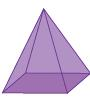Ans.

(i)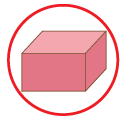(ii)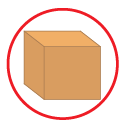(iii)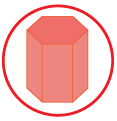(iv)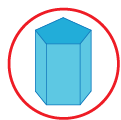Q.2. Pick the 3D shapes that slide.
(i)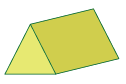(ii)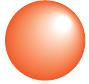(iii)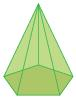(iv)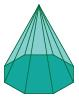(v)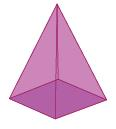(vi)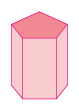Ans.

(i)(ii)(iii)(iv)(v)Q.3. Find out the 3D shapes that roll
(i)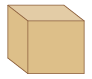(ii)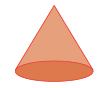(iii)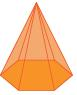(iv)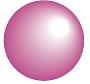(v)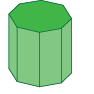(vi)Ans.

(i)(ii)(iii)Q.4. Fill in the blanks: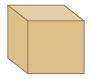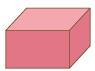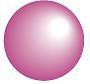(i) The cube and the cuboid each has _________ plane surfaces, _________ edges, and _________ vertices.
(ii) The cuboid has _________ pairs of identical plane surfaces.
(iii) All the surfaces of a cube are _________ to each other.
(iv) All the edges of a cube are _________.
(v) Opposite edges of a cuboid are _________.
(vi) A _________ has no vertex, and no edge.
Ans.

(i) 6, 12, 8
(ii) 3
(iii) equal line
(iv) straight lines
(v) same in size
(vi) sphere

Q.5.Write ‘true’ or ‘false’ for each statement as the case may be: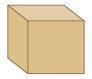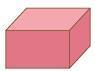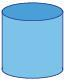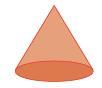(i) Every cuboid is a cube.
(ii) Every cube is a cuboid.
(iii) All faces of a cube are similar in shape and size.
(iv) All the faces of a cuboid are identical.
(v) The cube has 6 faces.
(vi) The base and bottom of a cylinder are identical.
(vii) A cone has no vertex.
(viii) A cylinder has two edges.
(ix) A sphere has no edge.
(x) A cone has two surfaces.
Ans.

(i) False
(ii) True
(iii) True
(iv) False
(v) True
(vi) True
(vii) False
(viii) True
(ix) True
(x) True

Offer running on EduRev: Apply code STAYHOME200 to get INR 200 off on our premium plan EduRev Infinity!

,

,

,

,

,

,

,

,

,

,

,

,

,

,

,

,

,

,

,

,

,

,

,

,

;